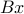# ASP – A Matlab solver for sparse optimization

ASP is a set of Matlab routines for solving several variations of the sparse optimization problem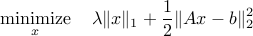It implements algorithm for the following:

• basis pursuit denoising (including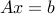)

• orthogonal matching pursuit

• homotopy version of basis pursuit denoising

• reweighted basis pursuit for approximating 0-norm solutions

• sequential compressed sensing (adding rows to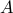and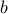)

• nonnegative least-squares

• sparse-residual and sparse-solution regression

• generalized Lasso for sparsity in